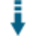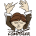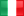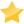# PHP Sun Position Calculator: Calculate Sun position astronomical distanceDownload .zip
 InfoExampleView files (19)Download .zip Reputation Support forum (1) Blog Links
 Ratings Unique User Downloads Download Rankings Not yet rated by the users Total: 161 All time: 8,916 This week: 257Version License PHP version Categories sun-position-spa-php 1.0.1 Custom (specified... 5 Algorithms, PHP 5, Physics
Collaborate with this project

Author

Description

This package can calculate the Sun position based on observations.

It takes as parameters the observer latitude, longitude and altitude, date, time and timezone, air pressure and temperature.

Then it can calculate the Sun position and returns the sunrise, sunset and transit.

It is a library based on the work of Ibrahim Reda and Afshin Andreas (SPA) Solar Position Algorithm for Solar Radiation Applications ( 2008 National Renewable Energy Laboratory ).Performance Level
Name: Classes: Francesco Danti `` 7 packages by Francesco DantiItaly 45 3505 140 in Italy40217 in ItalyLevel 1Innovation awardNominee: 2x

Details

# Sun-Position-SPA-php

SPA Sun Position Calc Library for PHP

This library is based on the work of Ibrahim Reda and Afshin Andreas (SPA) Solar Position Algorithm for Solar Radiation Applications ( 2008 National Renewable Energy Laboratory )

## Abstract from the original study

There have been many published articles describing solar position algorithms for solar radiation applications. The best uncertainty achieved in most of these articles is greater than �0.01 / in calculating the solar zenith and azimuth angles. For some, the algorithm is valid for a limited number of years varying from 15 years to a hundred years. This report is a step by step procedure for implementing an algorithm to calculate the solar zenith and azimuth angles in the period from the year -2000 to 6000, with uncertainties of �0.0003�

### PHPUNIT TEST

Library test data vs Table A.4 of the original study

### C Source code for Solar Position Algorithm (SPA)

http://www.nrel.gov/midc/spa/

### Requirements

- PHP 5.6 - PHP 7

### Composer install

``````composer require abbadon1334/sun-position-spa-php
``````

### Simple Usage

``````\$SD = new SolarData\SolarData();

/ARGS : observer latitude, observer longitude, observer altitude/
\$SD->setObserverPosition(39.742476,-105.1786,1830.14);

/ARGS : Observer Date : Year, Month, Day/
\$SD->setObserverDate(2003, 10, 17);

/ARGS : Observer Time : Hours, Minutes, Seconds/
\$SD->setObserverTime(12, 30,30);

/ARGS : difference in seconds between the Earth rotation time and the Terrestrial Time (TT)/
\$SD->setDeltaTime(67);
/ARGS : Observer Timezone/
\$SD->setObserverTimezone(-7);

/ARGS : Observer mean pressure in Millibar/
\$SD->object->setObserverAtmosphericPressure(820);

/ARGS : Observer mean temperature in Celsius/
\$SD->object->setObserverAtmosphericTemperature(11.0);

/calculate sun position/
\$SunPosition = \$SD->calculate();
``````

#### Available attributes after calculate() :

I know this attributes names are not so ortodox. Formulas that are present in the original document are really complex and using the same name for variables is a big aid for debugging

• `L�` Earth heliocentric longitude (degrees)
• `B�` Earth heliocentric latitude (degrees)
• `R` Earth radius vector, R (in Astronomical Units, AU)
• `?�` geocentric longitude (degrees)
• `?�` geocentric longitude (degrees)
• `X` nutation in longitude and obliquity
• `?�` true obliquity of the ecliptic (degrees)
• `??` aberration correction (degrees)
• `?�` apparent sun longitude (degrees)
• `?�` apparent sidereal time at Greenwich (degrees)
• `?0�` apparent mean sidereal time at Greenwich (degrees)
• `?�` geocentric sun right ascension (degrees)
• `?��` topocentric sun right ascension (degrees)
• `?�` geocentric sun declination (degrees)
• `?��` topocentric sun declination (degrees)
• `H�` Observer hour angle (degrees)
• `H��` topocentric hour angle (degrees)
• `?�` equatorial horizontal parallax of the sun (degrees)
• `Z�` topocentric zenith angle (degrees)
• `?�` topocentric astronomers azimuth angle (degrees)
• `?�` topocentric azimuth angle, M for navigators and solar radiation users (in degrees)
• `e0�` topocentric elevation angle without atmospheric refraction (in degrees)
• `e�` topocentric elevation angle (in degrees)
• `Eot` Equation Of Time

Example to get angle H� - Observer hour angle (degrees)

``````\$SD = new SolarData\SolarData();

/ARGS : observer latitude, observer longitude, observer altitude/
\$SD->setObserverPosition(39.742476,-105.1786,1830.14);

/ARGS : Observer Date : Year, Month, Day/
\$SD->setObserverDate(2003, 10, 17);

/ARGS : Observer Time : Hours, Minutes, Seconds/
\$SD->setObserverTime(12, 30,30);

/ARGS : difference in seconds between the Earth rotation time and the Terrestrial Time (TT)/
\$SD->setDeltaTime(67);
/ARGS : Observer Timezone/
\$SD->setObserverTimezone(-7);

/ARGS : Observer mean pressure in Millibar/
\$SD->object->setObserverAtmosphericPressure(820);

/ARGS : Observer mean temperature in Celsius/
\$SD->object->setObserverAtmosphericTemperature(11.0);

/calculate sun position/
\$SunPosition = \$SD->calculate();
``````

to get H� Observer hour angle (degrees)

``````echo \$SunPosition->H�;
``````

Example to get fraction day for sunrise - transit - sunset

``````
\$SD = new SolarData\SolarData();

/ARGS : observer latitude, observer longitude, observer altitude/
\$SD->setObserverPosition(39.742476,-105.1786,1830.14);

/ARGS : Observer Date : Year, Month, Day/
\$SD->setObserverDate(2003, 10, 17);

/ARGS : Observer Time : Hours, Minutes, Seconds/
\$SD->setObserverTime(12, 30,30);

/ARGS : difference in seconds between the Earth rotation time and the Terrestrial Time (TT)/
\$SD->setDeltaTime(67);
/ARGS : Observer Timezone/
\$SD->setObserverTimezone(-7);

/ARGS : Observer mean pressure in Millibar/
\$SD->object->setObserverAtmosphericPressure(820);

/ARGS : Observer mean temperature in Celsius/
\$SD->object->setObserverAtmosphericTemperature(11.0);

/* calculate sun position and calculate sun rise transit set angles
ARGS : true = call ->calculate()
*/
\$SunPosition = \$SD->calculateSunRiseTransitSet(true);

\$SunRiseDayFraction = \$SunPosition->DayFractionSunrise;
\$TransitDayFraction = \$SunPosition->DayFractionTransit;
\$SunsetDayFraction  = \$SunPosition->DayFractionSunset;

``````

### Get Sun Incidence Angle

``````
\$SD = new SolarData\SolarData();

/ARGS : observer latitude, observer longitude, observer altitude/
\$SD->setObserverPosition(39.742476,-105.1786,1830.14);

/ARGS : Observer Date : Year, Month, Day/
\$SD->setObserverDate(2003, 10, 17);

/ARGS : Observer Time : Hours, Minutes, Seconds/
\$SD->setObserverTime(12, 30,30);

/ARGS : difference in seconds between the Earth rotation time and the Terrestrial Time (TT)/
\$SD->setDeltaTime(67);
/ARGS : Observer Timezone/
\$SD->setObserverTimezone(-7);

/ARGS : Observer mean pressure in Millibar/
\$SD->object->setObserverAtmosphericPressure(820);

/ARGS : Observer mean temperature in Celsius/
\$SD->object->setObserverAtmosphericTemperature(11.0);

``````
• ``````no need of calling calculate *
/ARGS : tilt angle from horizontal plane, rotation angle from real south/
\$Surface2SunAngleOfIncidence = \$SD->getSurfaceIncidenceAngle(30,-10)
``````Files
File Role Descriptionsrc (2 files, 1 directory)tests (1 file).codeclimate.yml Data Auxiliary data.coveralls.yml Data Auxiliary data.phpmetrics.json Data Auxiliary data.php_cs.dist Example Example script.styleci.yml Data Auxiliary data.travis.yml Data Auxiliary datacomposer.json Data Auxiliary dataindex.php Example Example scriptLICENSE Lic. License textphpunit.xml Data Auxiliary dataREADME.md Doc. Documentationrenovate.json Data Auxiliary dataFiles / src
File Role DescriptionObserver (4 files)SolarData.php Class Class sourceSunPosition.php Class Class sourceFiles / src / Observer
File Role DescriptionObserver.php Class Class sourceObserverPosition.php Class Class sourceObserverTime.php Class Class sourceObserverWeather.php Class Class sourceFiles / tests
File Role DescriptionSolarDataTest.php Class Class source

 All time: 8,916 This week: 257About usAdvertise on this siteSite mapNewsletterStatisticsSite tipsPrivacy policyContact
For more information send a message to `info at phpclasses dot org`.